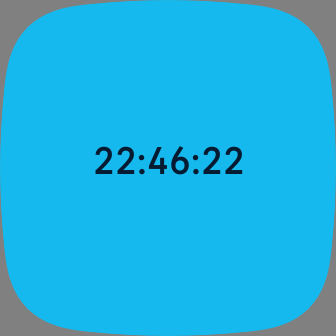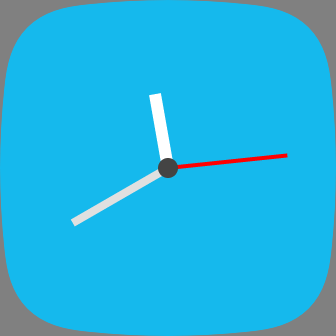Clock face Guide# Clock Face Guide

## Overview

The Clock API allows developers to easily update their app's display based on the current date and time.

The `tick` event contains a JavaScript `Date` object with the current date and time. Although `setInterval()` could be used in a similar fashion, the Clock API automatically handles tick events at the correct precision and timing.

``````import clock from "clock";

clock.granularity = "minutes"; // seconds, minutes, or hours

// tick every minute
});
``````

Another advantage of using the Clock API is that `tick` events do not occur when the display is off, so you're automatically reducing your impact upon battery life when using it.

## Digital Clock

To create a simple digital clock, we need a `<text>` component, and use the `tick` event to update the display.``````<svg viewport-fill="fb-cyan">
<text id="clock-label" x="50%" y="50%+15"
fill="black" font-size="32" font-family="System-Regular"
text-anchor="middle" text-length="20">00:00:00</text>
</svg>
``````

Then use JavaScript to update the `<text>` element every time the clock ticks.

``````import clock from "clock";
import * as document from "document";

clock.granularity = "seconds"; // seconds, minutes, hours

const clockLabel = document.getElementById("clock-label");

clockLabel.text = evt.date.toTimeString().slice(0, -4);
});
``````

## Analog Clock

The Clock API can also be used to easily create an analog clock. We take the current time from JavaScript, and use that to calculate the angles required to rotate hands.First, we define `<rect>` elements to use for the hour, minute and seconds, and position them from the center of the screen, pointing at the 12 o'clock position. We define a `<g>` elements for each hand, so they can be rotated independently of the other elements.

``````<svg viewport-fill="fb-cyan">
<g id="mins" pointer-events="visible" transform="translate(50%,50%)">
<rect x="\$-4" y="-110" width="8" height="110" fill="#e0e0e0" />
</g>
<g id="hours" pointer-events="visible" transform="translate(50%,50%)">
<rect x="\$-6" y="-75" width="12" height="75" fill="#ffffff" />
</g>
<g id="secs" pointer-events="visible" transform="translate(50%,50%)">
<rect x="\$-2" y="-120" width="4" height="120" fill="#ff0000" />
</g>
<circle cx="50%" cy="50%" r="10" fill="#444444" />
</svg>
``````
``````import clock from "clock";
import * as document from "document";

// Tick every second
clock.granularity = "seconds";

let hourHand = document.getElementById("hours");
let minHand = document.getElementById("mins");
let secHand = document.getElementById("secs");

// Returns an angle (0-360) for the current hour in the day, including minutes
function hoursToAngle(hours, minutes) {
let hourAngle = (360 / 12) * hours;
let minAngle = (360 / 12 / 60) * minutes;
return hourAngle + minAngle;
}

// Returns an angle (0-360) for minutes
function minutesToAngle(minutes) {
return (360 / 60) * minutes;
}

// Returns an angle (0-360) for seconds
function secondsToAngle(seconds) {
return (360 / 60) * seconds;
}

// Rotate the hands every tick
function updateClock() {
let today = new Date();
let hours = today.getHours() % 12;
let mins = today.getMinutes();
let secs = today.getSeconds();

hourHand.groupTransform.rotate.angle = hoursToAngle(hours, mins);
minHand.groupTransform.rotate.angle = minutesToAngle(mins);
secHand.groupTransform.rotate.angle = secondsToAngle(secs);
}

// Update the clock every tick event